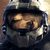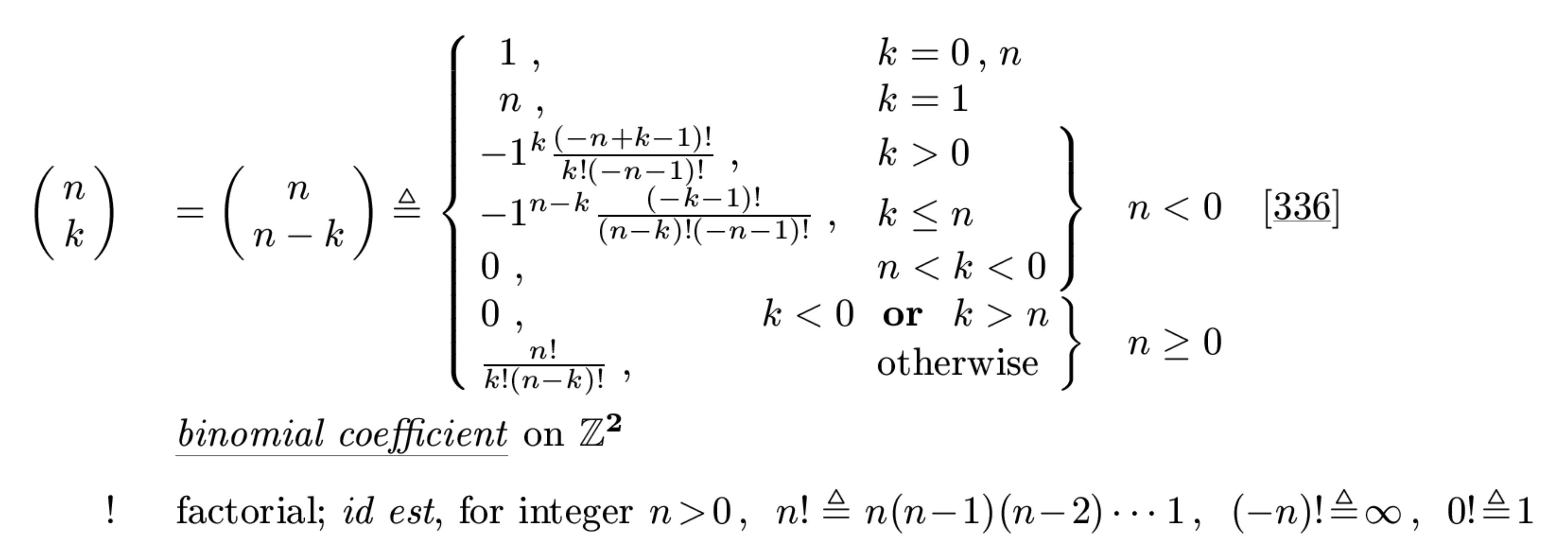0

# nchoosek, binomial coefficient

dattorro 3 years ago updated 2 years ago

How do we increase dynamic range of Matlab's binomial coefficient function nchoosek()?

It will not accept multiprecision mp() input.Under review

Toolbox doesn't include this function at the moment. Now I am considering to add it.

How do you use it? Just to compute binomial coefficient or to generate all combinations?There is a quick way to make the built-in 'nchoosek()' work with toolbox. Create flintmax.m file in [toolbox_folder]\@char directory with following content:

```function r = flintmax( classname )
if strcmpi('mp',classname)        r = 2^ceil(mp.Digits() * mp('log2(10)'));    else        r = builtin('flintmax',classname);    endend```

Restart MATLAB and test 'nchoosek()', e.g.:

```>> mp.Digits(100);
>> x = nchoosek(mp(1000),50)

x =

9460461017585217574825413104128279942327590136138287606176460370151247948488653144064```We have just updated toolbox distribution/installer with the 'flintmax.m' already included.